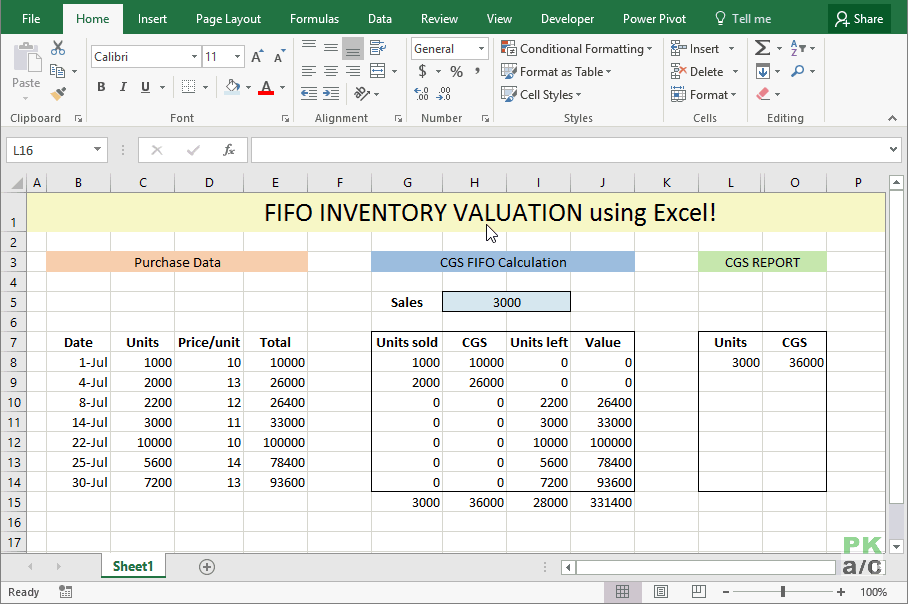FIFO Inventory Valuation in Excel – Step by Step - KING OF EXCEL

## Friday, August 18, 2023FIFO Inventory Valuation in Excel – Step by Step

Inventory can be valued in number of ways, FIFO, LIFO and AVCO being the most famous. To learn few more inventory valuation methods have a quick look at this: What are different inventory valuation methods?Entities purchase inventory as and when they feel the need or based on a particular method for example Economic Order Quantity (EOQ). Often the the purchase price is different every time order is placed. Therefore, the price of each purchase lot is different from each other as you can see in the data at left

First In First Out (FIFO) assumes that every time units are taken from store, they are issued from the oldest available lot first and next lot to be consumed only if needed.

This requirement makes the calculations a little too complex for formulas. May be there is a way to do it via formulas but I am unaware of it yet and would love to know. On the other hand VBA is also an alien knowledge for me so far. So is there a way? Yes there is!

By the end of the tutorial we will be able to get this:This is where Excel Data Tables comes handy. One of the less known and used tool for sure. But it can make this process super smooth and easy. So lets get to learn it!

Important: Excel Data Tables are different from Excel Tables so please don’t confuse the two

FIFO Inventory Valuation in Excel – Step by Step

Step 1: Download this workbook. It has the dummy data that we can use to understand the process.

Step 2: Format the worksheet as follows so that we know exactly what information is where:Step 3: Suppose we have made a sale of 1000 units. So enter that in cell G1 and name the cell as “sales” by typing in the name box having cell G1 active.Step 4: Now we want the total units sold to be divided as per lots available deducting from the first available lot. Go to cell F4 and put this formula and drag it down to cell F10.

=MIN(B4,sales-SUM(\$F\$3:F3))

Step 5: Next put the following formulas in the mentioned cells and drag them to row 10:

G4: =F4*C4
H4: =B4-F4
I4: =H4*C4Step 6: Calculate the totals of each column by simply selecting the 11th row and hitting ALT+=So we have completed the calculations and if you change the value in sales we can easily update the records of each column showing the correct cost and value of inventory at handThough the above process has helped me get the numbers but its not helping much if I want to calculate the cost of sales for different quantities. For example if I want to know the cost of sales of all the quantities by the end of the month, I simply need a report instead of punching each quantity in cell and then record the value somewhere. To solve this we will take few more steps

Step 7: Format the cells as follows. This is where we will have a mini report generator for the units you mention and Excel will automatically calculate the CGS of units mentioned on FIFO basis:Step 8: In cell L4 put the following formula and drag the fill handle down to cell L10

=SUM(\$K\$4:K4)Step 9: In cell M3 refer to total of CGS we calculated before in cell G11 with this formula: =G11

Step 10: Select the range L3: M10 and go to Data tab > Forecast group > Click what-if drop down button > click Data table. A pop up will appear. Leave the row input cell empty and mention units sold in column input cell G1. Click OK.Step 11: Put the following formula in CGS column’s cells:

N4: =M4
N5: =M5-SUM(\$N\$4:N4), drag the fill handle down to cell N10Step 12: Select cells N4:N10 and hit Ctrl+1. Go to Custom and in the input bar mention the following and click OK:

0;0;

Step 13: Hide the columns L and M. Now test your report by typing the units in “Units” column and it will give you the correct CGS as per FIFO.So here you have fully working FIFO Calculator and a mini report generator!

### Liked it? Pin it!